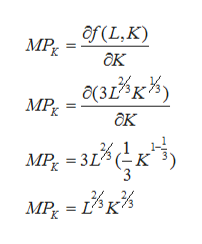# Consider a firm that has production function f(L,K)= 3L2/3K1/3. What is the expression for this firm’s Marginal Product of capital? MPK(L,K)= 3L2/3/K1/3.MPK(L,K)= 3L2/3/K2/3.MPK(L,K)= L1/3/K1/3.MPK(L,K)= L2/3/K2/3.MPK(L,K)= 2L2/3/K1/3.

Question
3 views

Consider a firm that has production function f(L,K)= 3L2/3K1/3. What is the expression for this firm’s Marginal Product of capital?

MPK(L,K)= 3L2/3/K1/3.

MPK(L,K)= 3L2/3/K2/3.

MPK(L,K)= L1/3/K1/3.

MPK(L,K)= L2/3/K2/3.

MPK(L,K)= 2L2/3/K1/3.

check_circle

Step 1

Firm’s production function is given as:

Step 2

Marginal product of capital is the increase in output of a firm when a unit of capital is added to production. ...help_outlineImage Transcriptioncloseof(L,K) MPx ак 28вк* MPR ок МP, — 313 (-к вВк% MP L K fullscreen

### Want to see the full answer?

See Solution

#### Want to see this answer and more?

Solutions are written by subject experts who are available 24/7. Questions are typically answered within 1 hour.*

See Solution
*Response times may vary by subject and question.
Tagged in